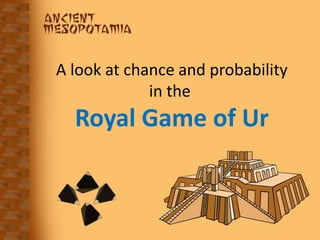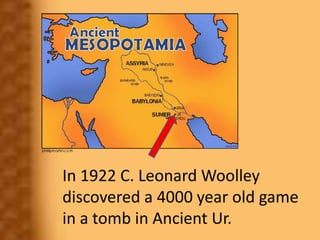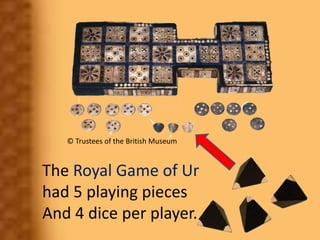Successfully reported this slideshow.

# Probability in and Ancient Game×

# Probability in and Ancient Game

This presentation looks at the dice used in the 4000 year old Royal Game of Ur discovered in the 1920's by C. Leonard Woolley in the ruins of the ancient city of Ur in Mesopotamia

This presentation looks at the dice used in the 4000 year old Royal Game of Ur discovered in the 1920's by C. Leonard Woolley in the ruins of the ancient city of Ur in Mesopotamia

### Probability in and Ancient Game

1. 1. A look at chance and probability in the Royal Game of Ur
2. 2. In 1922 C. Leonard Woolley discovered a 4000 year old game in a tomb in Ancient Ur.
3. 3. The Royal Game of Ur had 5 playing pieces And 4 dice per player. © Trustees of the British Museum
4. 4. Each die was a 4-sided equilateral pyramid. Two of the 4 corners where marked white. This meant that when a die was rolled, there were 2 chances in 4 of getting a white corner. 𝑃 𝑤ℎ𝑖𝑡𝑒 = 2 4 = 𝟏 𝟐
5. 5. So the probability of rolling 1 (if a white corner counts as a 1) is 𝑃 𝑤ℎ𝑖𝑡𝑒 = 2 4 = 𝟏 𝟐
6. 6. If a player has 4 pyramid dice to roll, then a 0, 1, 2, 3 or 4 could be rolled. Is there an EQUAL chance of these scores happening?
7. 7. We will draw a tree diagram to help us answer this question. A white corner will be represented by a W and a black corner with a B. Every white at the top after a throw counts as 1. W B This would count as 0.
8. 8. In the tree diagram there will be 4 events matching the results of the 4 dice thrown. W B First dice 𝑃 𝑤ℎ𝑖𝑡𝑒 = 2 4 = 𝟏 𝟐 𝑃 𝑏𝑙𝑎𝑐𝑘 = 2 4 = 𝟏 𝟐 1 2 1 2 So the chance of rolling a 1 (a white tip) is 1 chance in 2.
9. 9. W W B B W B 1st dice 2nd dice 1 2 1 2 1 2 1 2 1 2 1 2 When the 2nd pyramid is rolled, once again there is a 1 chance in 2 of getting Another white tip. The chance of getting two white tips (rolling a 2) is found by multiplying along the branch of the tree diagram. This means that the chance of rolling a 2 is This is 1 chance in 4. × 1 2 × 1 2 = 𝟏 𝟒
10. 10. W W B B W B 1st dice 2nd dice 1 2 1 2 1 2 1 2 1 2 1 2 The same can be done for each branch of the tree. × Probability of rolling a 2 is 1 4 Probability of rolling a 1 is 1 4 Probability of rolling a 1 is 1 4 Probability of rolling a 0 is 1 4
11. 11. W W B B W B 1st dice 2nd dice 1 2 1 2 1 2 1 2 1 2 1 2 The same can be done for each branch of the tree. × Probability of rolling a 2 is 1 4 Probability of rolling a 1 is 1 4 Probability of rolling a 1 is 1 4 Probability of rolling a 0 is 1 4 Since there are two possibilities of rolling a 1, we add the fractions. This gives a 2 4 or 1 2 chance of rolling a 1. The combined probability of rolling a 1 is 1 4 + 1 4 = 1 2
12. 12. Here is the tree diagram for rolling the 4 pyramid dice. 1st die 2nd die 3rd die 4th die
13. 13. W B Here is the tree diagram for rolling the 4 pyramid dice. 1st die 2nd die 3rd die 4th die
14. 14. W W B B W B Here is the tree diagram for rolling the 4 pyramid dice. 1st die 2nd die 3rd die 4th die
15. 15. W W B W B W B B W B W B W B Here is the tree diagram for rolling the 4 pyramid dice. 1st die 2nd die 3rd die 4th die
16. 16. W W B W B W B W B W B W B W B B W B W B W B W B W B W B W B Here is the tree diagram for rolling the 4 pyramid dice. 1st die 2nd die 3rd die 4th die
17. 17. Since each arrow has a probability of 1 2 and we multiply along a branch to find the chance of that branch occurring, each branch line has a probability of 1 2 × 1 2 × 1 2 × 1 2 = 1 16 . Follow 2 branch lines to see how this happens.
18. 18. 1st die 2nd die 3rd die 4th die 𝟏 𝟐
19. 19. W W B 1st die 2nd die 3rd die 4th die 𝟏 𝟐 𝟏 𝟐 𝟏 𝟐 × ×
20. 20. W W B W B W B 1st die 2nd die 3rd die 4th die 𝟏 𝟐 𝟏 𝟐 𝟏 𝟐 𝟏 𝟐 𝟏 𝟐 × × × ×
21. 21. W W B W B W B W B W B W B W B 1st die 2nd die 3rd die 4th die 𝟏 𝟐 𝟏 𝟐 𝟏 𝟐 𝟏 𝟐 𝟏 𝟐 𝟏 𝟐 𝟏 𝟐 × × × × × ×
22. 22. W W B W B W B W B W B W B W B 1st die 2nd die 3rd die 4th die 𝟏 𝟐 𝟏 𝟐 𝟏 𝟐 𝟏 𝟐 𝟏 𝟐 𝟏 𝟐 𝟏 𝟐 × × × × = 𝟏 𝟏𝟔 × × = 𝟏 𝟏𝟔
23. 23. W W B W B W B W B W B W B W B 1st die 2nd die 3rd die 4th die 𝟏 𝟐 𝟏 𝟐 𝟏 𝟐 𝟏 𝟐 𝟏 𝟐 𝟏 𝟐 𝟏 𝟐 × × × × = 𝟏 𝟏𝟔 × × = 𝟏 𝟏𝟔 The probability of each branch occurring can be written like this: P(W, W, W, W) = 1 16 P(W, B, B, B) = 1 16 This can be done for each branch of the tree.
24. 24. Only one branch of the tree will give a roll of 4. So the chance of rolling a 4 will always be 1 16 . W W B W B W B W B W B W B W B B W B W B W B W B W B W B W B 1st die 2nd die 3rd die 4th die
25. 25. But what about the chance of rolling a 2? There are 6 branches that have 2 whites (a score of 2). W W B W B W B W B W B W B W B B W B W B W B W B W B W B W B 1st die 2nd die 3rd die 4th die 1 16 1 16 1 16 1 16 1 16 1 16
26. 26. But what about the chance of rolling a 2? There are 6 branches that have 2 whites (a score of 2). To find the probability of rolling a 2, add the chances of each of the 6 branches. W W B W B W B W B W B W B W B B W B W B W B W B W B W B W B 1st die 2nd die 3rd die 4th die 1 16 1 16 1 16 1 16 1 16 1 16
27. 27. The chance of rolling a score of 2 is: 1 16 + 1 16 + 1 16 + 1 16 + 1 16 + 1 16 = 6 16 W W B W B W B W B W B W B W B B W B W B W B W B W B W B W B 1st die 2nd die 3rd die 4th die 1 16 1 16 1 16 1 16 1 16 1 16
28. 28. By doing this for each of the possible outcomes (0, 1, 2, 3 or 4) the probabilities for each option turn out to be: P(0) = 1 16 P(1) = 4 16 P(2) = 6 16 P(3) = 4 16 P(4) = 1 16
29. 29. So our original question was: Is there an EQUAL chance of these scores happening? The answer: NO!
30. 30. Now see if knowing these probabilities will help you in your game. Go to the link below to the British Museum website and play the Royal Game of Ur online. (Requires Shockwave) © Trustees of the British Museum http://www.mesopotamia.co.uk/tombs/challenge/cha_set.html